## 前言

《笑傲江湖》中的“剑宗余孽”封不平本想仗着有嵩山派撑腰，一举夺了华山掌门宝座。可打了半天剑法上占不了便宜，最后只能使出“狂风快剑”，企图以内力取胜。可见，任何高明武功若无内功心法相辅，也是徒劳无功。

## 开闭原则

• Single Responsibility Principle 单一责任原则
• The Open Closed Principle 开放封闭原则
• The Liskov Substitution Principle 里氏替换原则
• The Dependency Inversion Principle 依赖倒置原则
• The Interface Segregation Principle 接口分离原则
SOLID 五大原则的出发点也是软件工程的终极目标：“高内聚、低耦合”。在后端开发中运用最多的是“依赖倒置原则”，与其相关的设计模式大约有5-6个。如下图所示：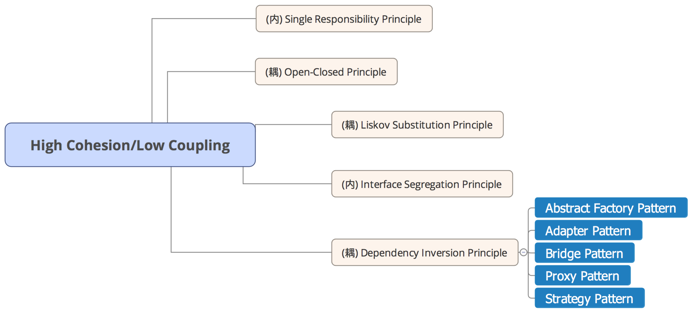A software artifact should be open for extension but closed for modification.

``````public abstract class Shape
{
public abstract double Area();
}

public class Rectangle: Shape
{
public double Width { get; set;}
public double Height { get; set;}
public override double Area()
{
return Width*Height'
}
}

public class Circle: Shape
{
public double Radius { get; set}
public override double Area()
{
}
}

public double Area(Shape [] shapes)
{
doubel area = 0;
foreach (var shape in shapes)
{
area += shape.Area();
}
return area;
}
``````

``````<FormItem>
})(<Input prefix={<Icon type="user" style={{ color: 'rgba(0,0,0,.25)' }} />}
)}
</FormItem>
``````

1. 提供受控属性 value 或其它与 valuePropName 的值同名的属性。
2. 提供 onChange 事件或 trigger 的值同名的事件。
3. 不能是函数式组件。

Ant Design 中的 Form 组件通过这样一个简洁的设计，完美提供了表单类型页面的统一解决方案。

## 函数式编程

• 函数为“一等公民”
• 模块化、组合
• 引用透明
• 避免状态改变
• 避免共享状态
JS 语言中的函数不同于 Java ，C/C++ 等语言, 可以被当做参数和返回值进行传递，因此天生具备“一等公民”特性。“模块化、组合”、“引用透明”、“避免状态改变”、“避免共享状态”这四个特征都需要通过特定代码模式实现。先举两个小例子：

### 找出字符串中率先出现的四个非数字字符？

##### 非 FP 风格
``````var words = [], count = 0;
var text = str.split('');
for (var i = 0; couont < 4, i < text.length; i++) {
if(!text[i].match(/[0-9]/)) {
words = words.concat(text[i]);
count++;
}
}
``````
##### FP 风格
``````var words = str.split('').filter(function(x){
return (!x.match(/[1-9]+/))}).slice(0,4);
``````

### 分别实现数组所有元素相加、相乘、相与？

##### 非 FP 风格
``````function plus(array) {
var res = array;
for (let i = 1; i < array.length; i++) {
res += array[i];
}
}

function mul(array) {
var res = array;
for (let i = 1; i < array.length; i++) {
res *= array[i];
}
}

function and (array) {
var res = array;
for (let i = 1; i < array.length; i++) {
res = res & array[i];
}
}

plus(array);
mul(array);
and(array);
``````
##### FP 风格
``````var ops = {
"plus": (x,y)=>x+y,
"mul" : (x,y)=>x*y,
"and" : (x,y)=>x&y
}

function operation(op, array) {
return array.slice(1).reduce(ops[op], array);
}

operation("plus", array);
operation("mul",  array);
operation("and",  array);
``````

### React 中的 FP 思想

React 框架中，当用户操作 UI 或者 API 的返回带来了数据的改变，React 随即进行 virtual dom diff 计算得到 dom 的修改指令，对 dom 元素应用修改指令便得到最新的 html 界面，如下图所示：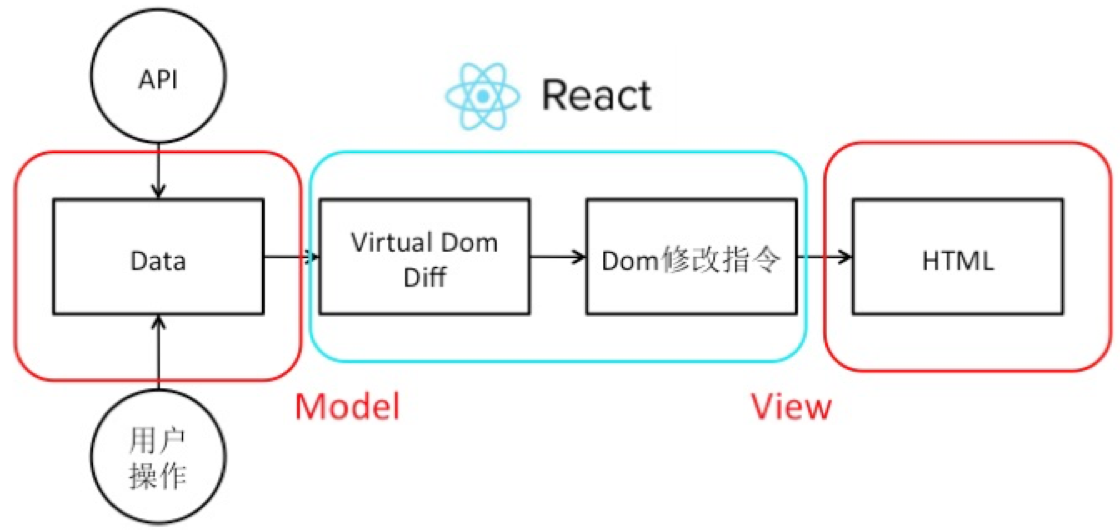\$UI = React(data)\$

## 消息机制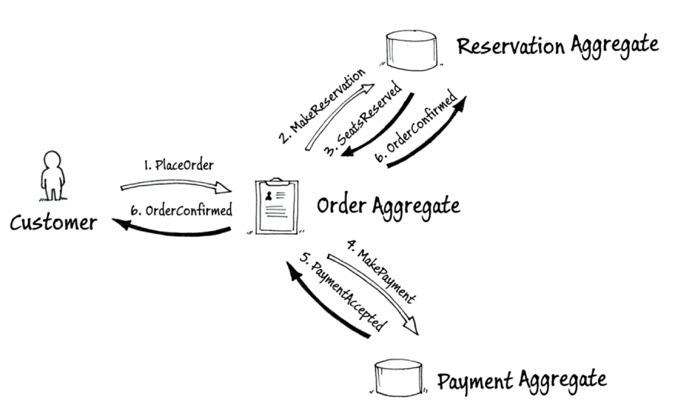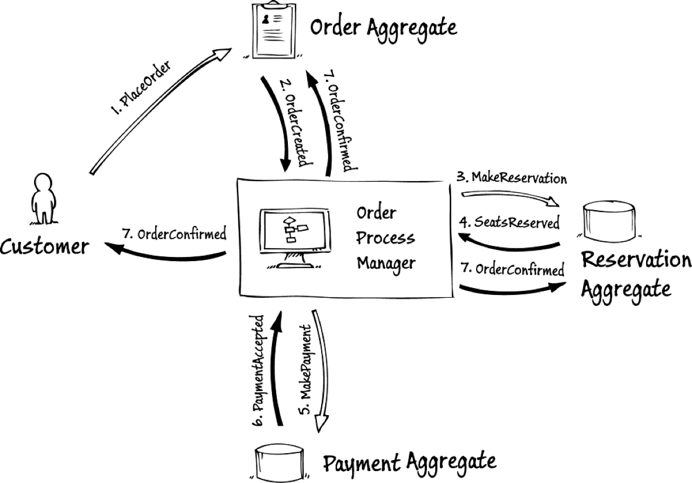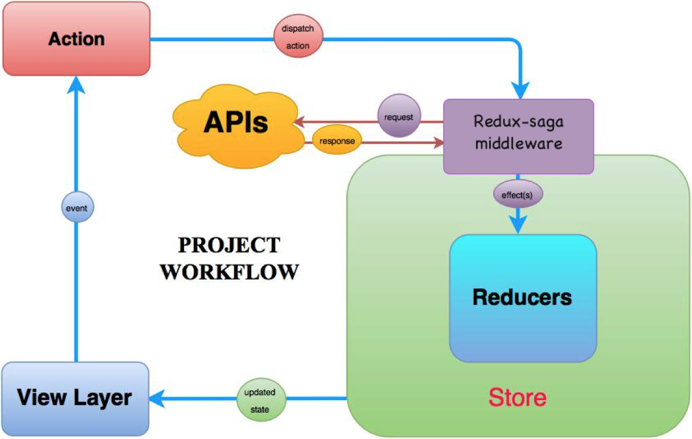## 总结

“开闭原则”、“函数式编程”、“消息机制”这个三个软件工程中重要的思想方法好比三套内功口诀，掌握了他们，才能更深刻地理解技术框架本身，发挥出技术框架的最大威力。